Examples

Chapter 9 Class 12 Differential Equations
Serial order wise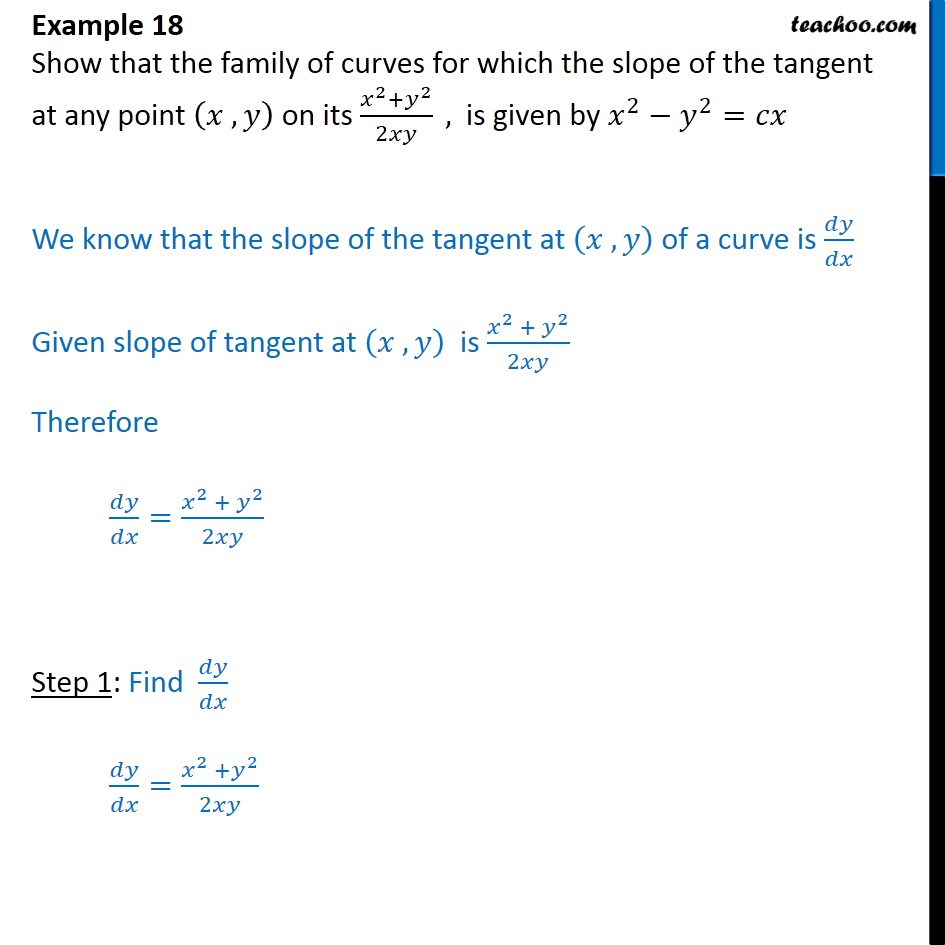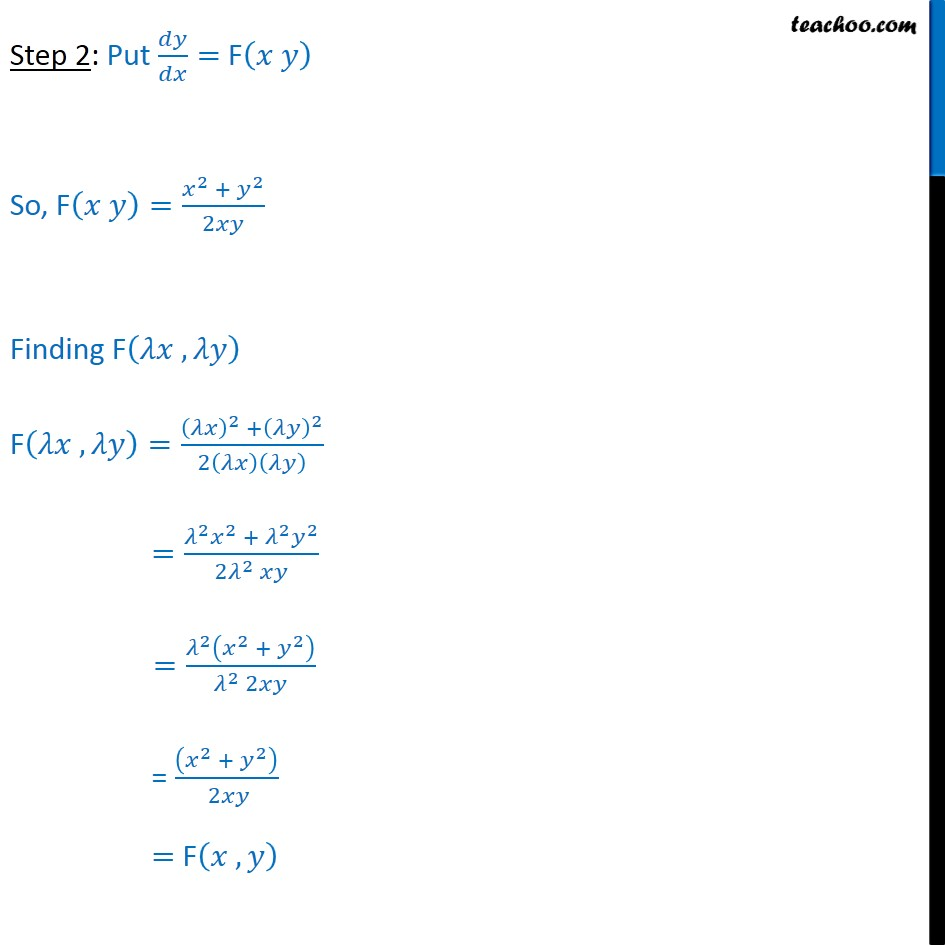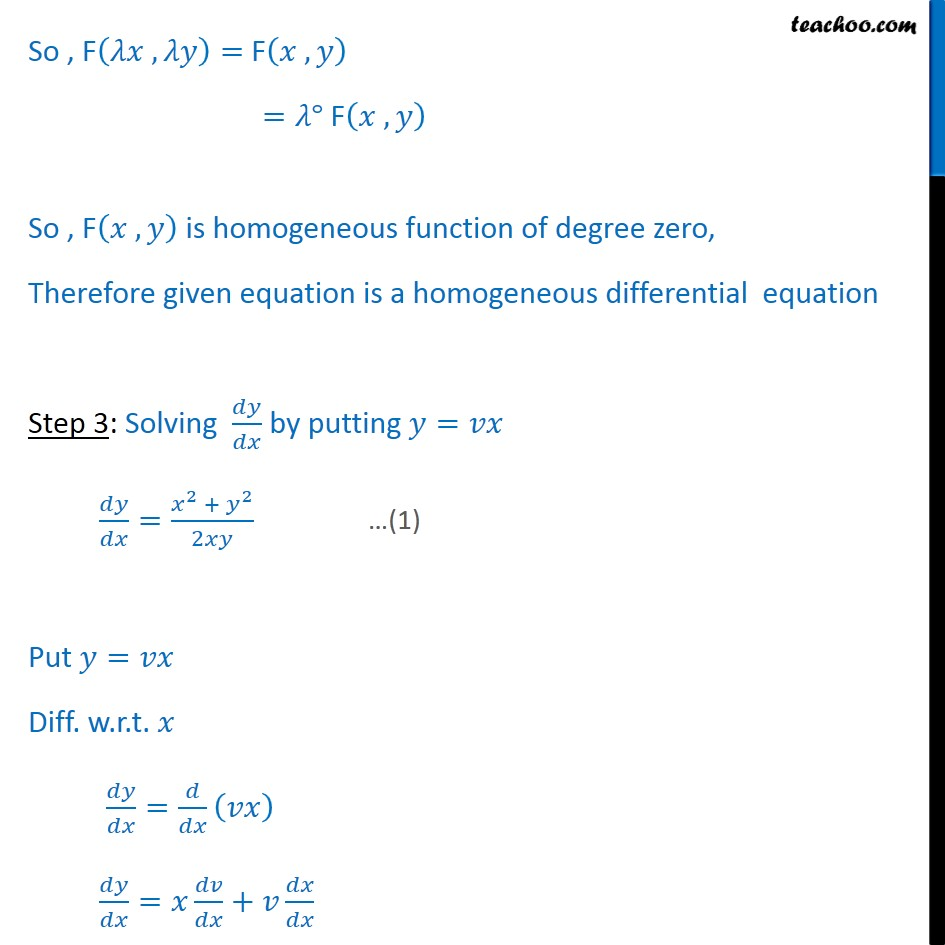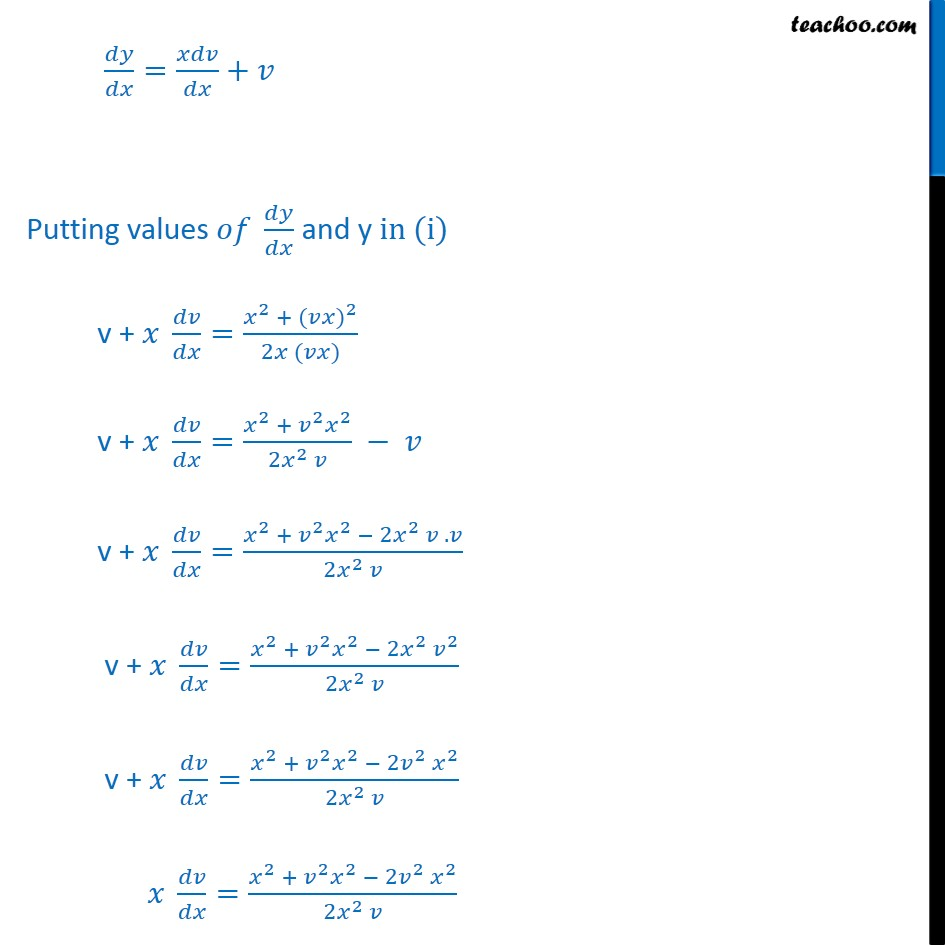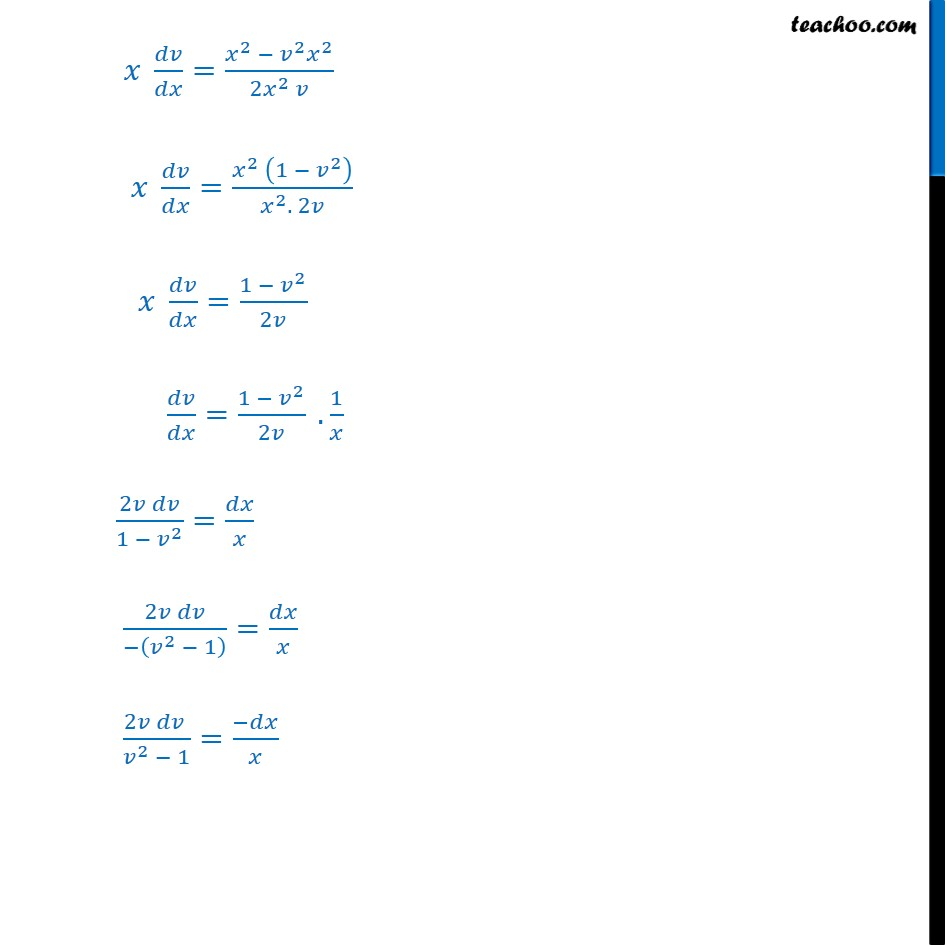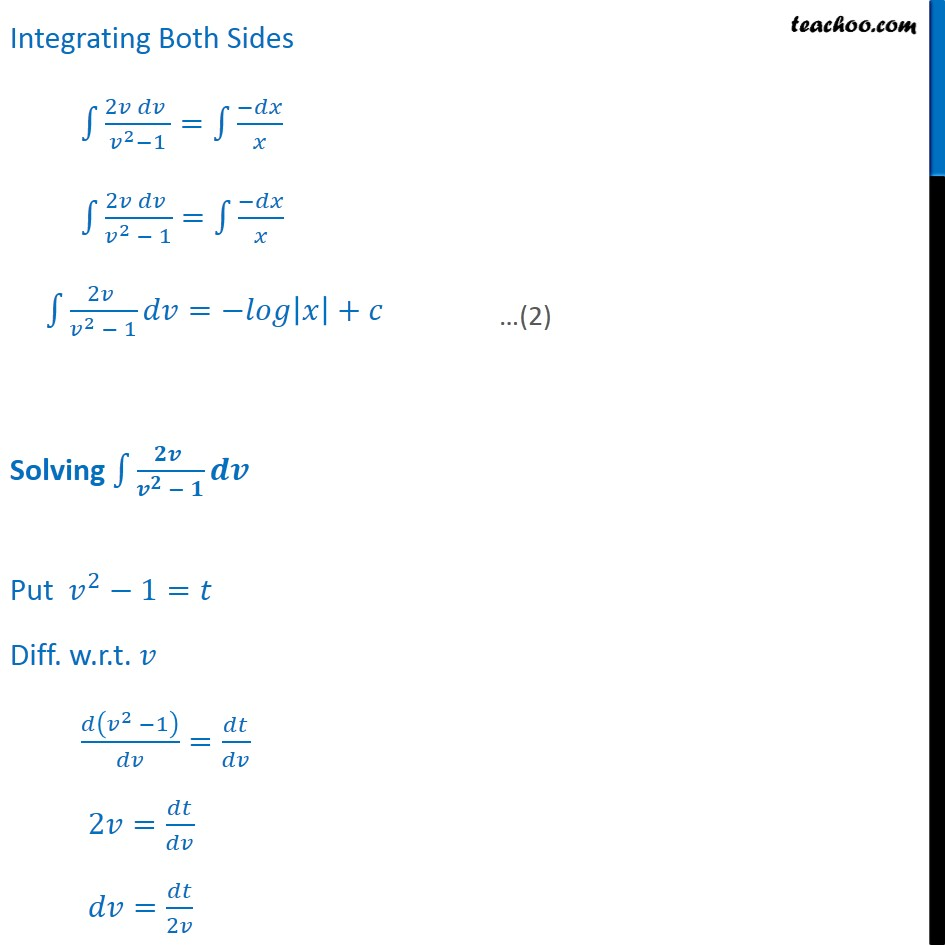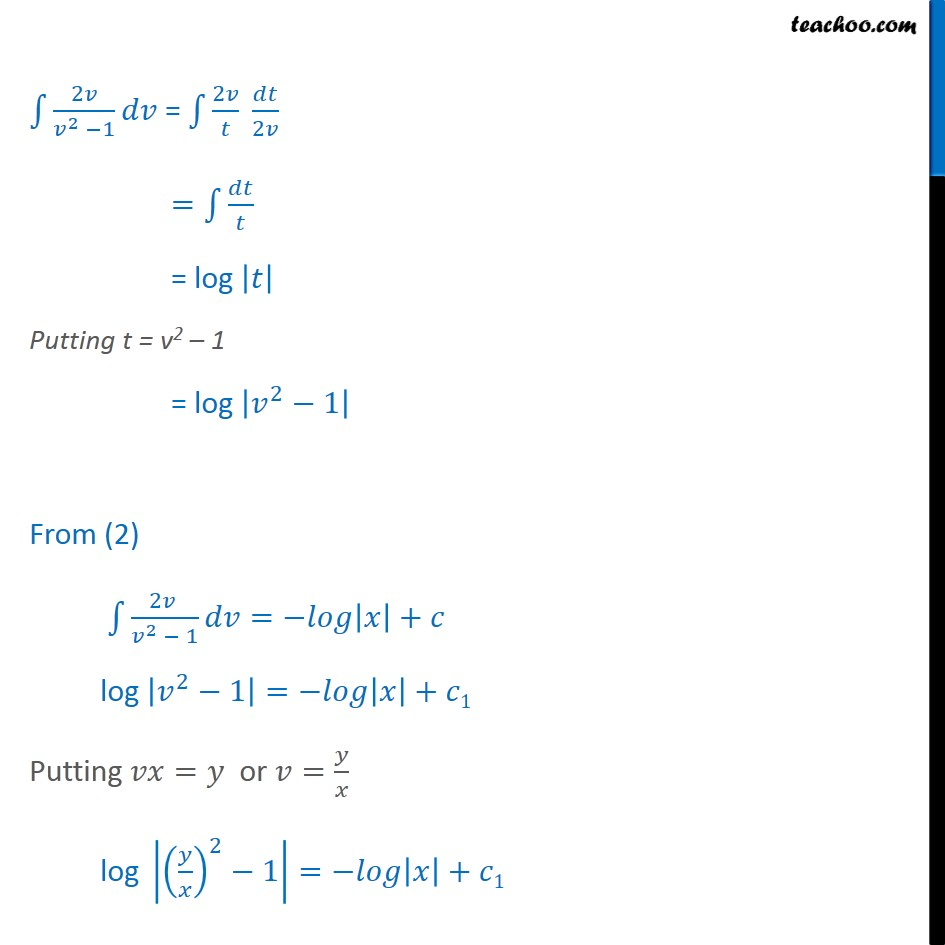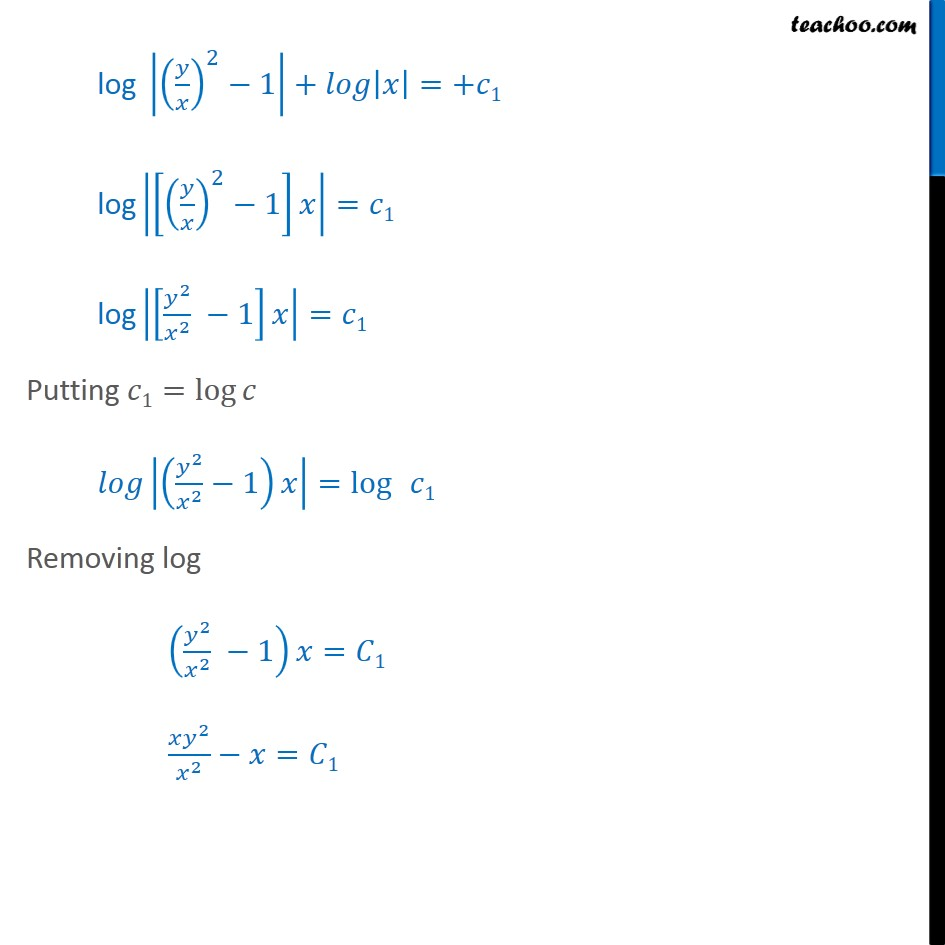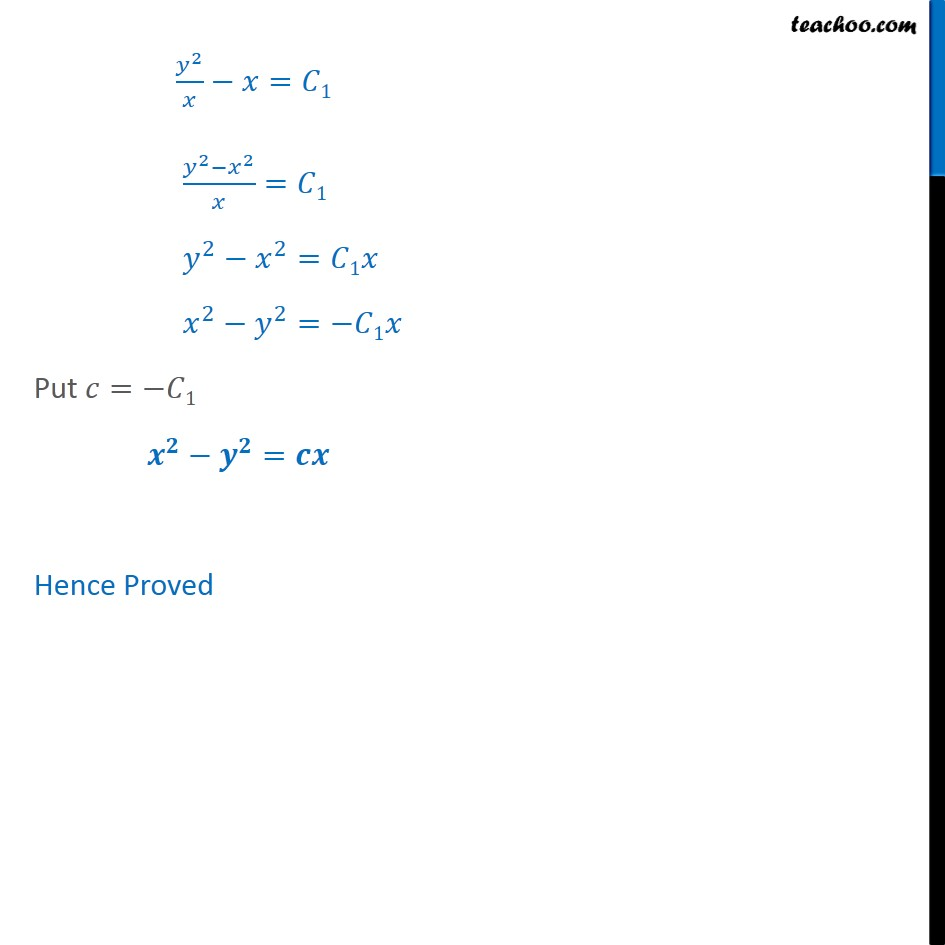Learn in your speed, with individual attention - Teachoo Maths 1-on-1 Class

### Transcript

Example 13 Show that the family of curves for which the slope of the tangent at any point , on its 2 + 2 2 , is given by 2 2 = We know that the slope of the tangent at , of a curve is Given slope of tangent at , is 2 + 2 2 Therefore = 2 + 2 2 Step 1: Find = 2 + 2 2 Step 2: Put = F So, F = 2 + 2 2 Finding F , F , = 2 + 2 2 = 2 2 + 2 2 2 2 = 2 2 + 2 2 2 = 2 + 2 2 = F , So , F , = F , = F , So , F , is homogeneous function of degree zero, Therefore given equation is a homogeneous differential equation Step 3: Solving by putting = = 2 + 2 2 Put = Diff. w.r.t. = = + = + Putting values and y in (i) v + = 2 + ( ) 2 2 ( ) v + = 2 + 2 2 2 2 v + = 2 + 2 2 2 2 . 2 2 v + = 2 + 2 2 2 2 2 2 2 v + = 2 + 2 2 2 2 2 2 2 = 2 + 2 2 2 2 2 2 2 = 2 2 2 2 2 = 2 1 2 2 . 2 = 1 2 2 = 1 2 2 . 1 2 1 2 = 2 2 1 = 2 2 1 = Integrating Both Sides 2 2 1 = 2 2 1 = 2 2 1 = + Solving Put 2 1= Diff. w.r.t. 2 1 = 2 = = 2 2 2 1 = 2 2 = = log Putting t = v2 1 = log 2 1 From (2) 2 2 1 = + log 2 1 = + 1 Putting = or = log 2 1 = + 1 log 2 1 = + 1 log 2 1 + =+ 1 2 1 = 1 2 2 1 = 1 Putting 1= log 2 2 1 = log 1 Removing log 2 2 1 = 1 2 2 = 1 2 = 1 2 2 = 1 2 2 = 1 2 2 = 1 Put = 1 = Hence Proved